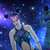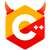# 2031. 绝对值排序### python water

while True:
try:
n = int(input())
L = list(input().split())
L = [int(e) for e in L]
L1 = [abs(e) for e in L]
for i in range(len(L1)):
for j in range(i+1,len(L1)):
if L1[i] >= L1[j]:
t = L1[i]
L1[i] = L1[j]
L1[j] = t
s = L[i]
L[i] = L[j]
L[j] = s
k = 0
while k < n-1:
print(L[k],end=” “)
k = k + 1
print(L[n-1])
except:
break#include <algorithm>
#include <cmath>
#include <cstdio>
#include <climits>

constexpr int NO_MORE = INT_MAX;
inline int get_int() {
int ans = 0;
bool fuvu = false;
signed char ch = getchar();
while (ch < '0' || '9' < ch) {
if (ch == '-')
fuvu = true;
if (ch == EOF)
return NO_MORE;
ch = getchar();
}
while ('0' <= ch && ch <= '9') {
ans = ans * 10 + ch - '0';
ch = getchar();
}
return fuvu ? -ans : ans;
}

int main() {
int n;
while ((n = get_int()) != NO_MORE) {
if (n == 0) {
putchar('\n');
continue;
}
int *const nums = new int[n], *const ed = nums + n;
for (int *i = nums; i != ed; ++i)
*i = get_int();
std::sort(nums, ed, [](int a, int b) { return abs(a) < abs(b); });
printf("%i", *nums);
for (int *i = nums + 1; i != ed; ++i)
printf(" %i", *i);
putchar('\n');
delete[] nums;
}
}